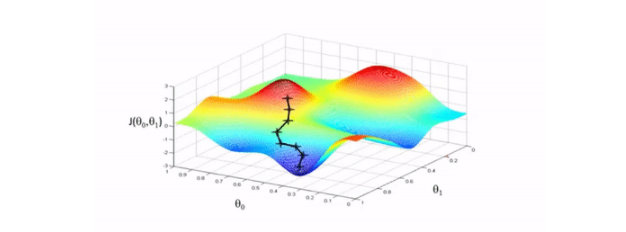python里反向传播算法是什么？# 实现 sigmoid 函数
return 1 / (1 + np.exp(-x))
def sigmoid_derivative(x):
# sigmoid 导数的计算
return sigmoid(x)*(1-sigmoid(x))

def relu_derivative(x): # ReLU 函数的导数
d = np.array(x, copy=True) # 用于保存梯度的张量
d[x < 0] = 0 # 元素为负的导数为 0
d[x >= 0] = 1 # 元素为正的导数为 1
return d

Python开发开发项目